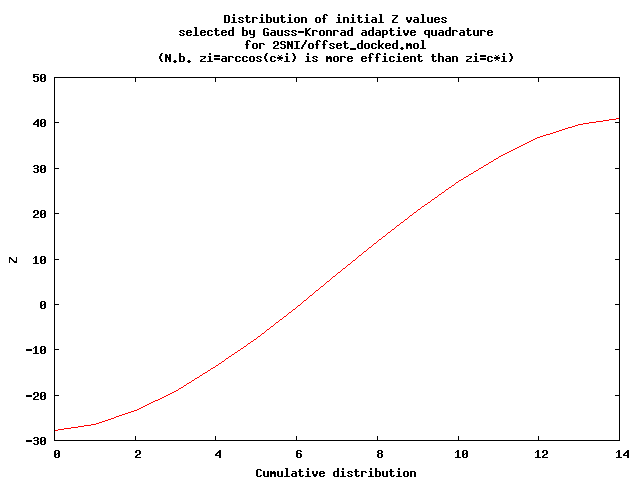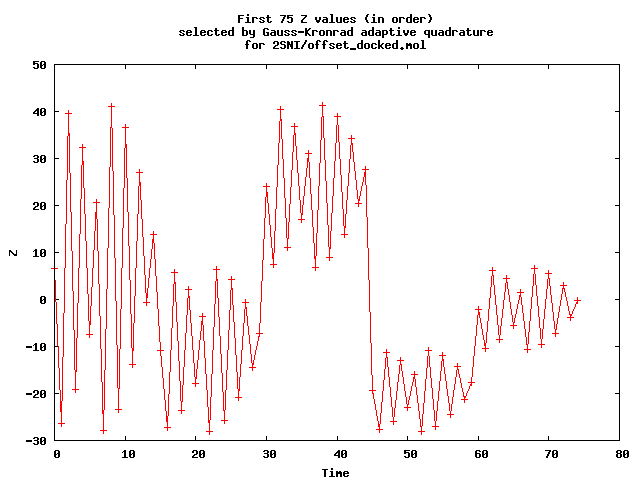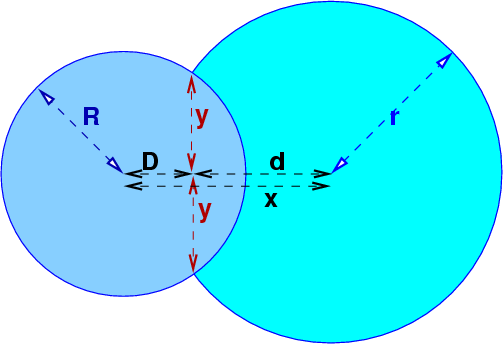See UniteCircles

# Intro

We wish to find the area of the surface of the union of intersecting spheres. The application is computing the solvent accessible area of a protein.

The surface may be partitioned into regions that are not exactly spherical triangles. Each region has 3 sides: arcs of 2 great circles, and an arc of a small circle. An example of a small circle is a parallel of latitude that is not the equator or a pole.

Unfortunately it appears that (except for special cases) the area of such a region has no closed form in terms of the usual elementary functions. That statement is based on my failure to integrate a simple special case in Maple. If this appearance is wrong, then great. Until then, the area must be approximated.

This raises the question of where in the computation process should the approximation occur. Here are some possibilities.

1. Approximate each sphere as a union of (possibly intersecting) cubes, and use our algorithm and implementation for the area of the union of cubes, which has been tested on 20M cubes.
However, since the cubes' surface normals don't approach the spheres', neither would the area. Also, if we want to go this route, octrees, i.e., nonintersecting cubes, would be simpler.
2. Approximate each sphere by a polyhedron, which is a tradition in Computer Graphics.
However, this uses an approximation in 2 dimensions, while we need to approximate in only 1. More approximation than necessary seems suboptimal.
3. Approximate the small circle's arc by a sequence of little pieces of great circles.
This would work, but the next idea is better.
4. Build upon our procedure in E2 for finding the perimeter of the union of circles, UniteCircles.
This is better is the earlier idea since it doesn't try to approximate each spherical non-triangle, but only their sum. The law of large numbers will be in our favor.

# The Method

1. There are N spheres.
2. si is the i-th sphere.
3. Compare each pair of spheres, to see whether they intersect. For each sphere, store a list of the other spheres intersecting it.
Note: Altho this takes quadratic time, that is still insignificant for our largest example so far (2451 spheres.)
4. Swap the molecule's X, Y, and Z coordinates to make its Z-extent larger than its X and Y-extents.
5. Prepare to slice the molecule on a sequence of parallel planes of constant Z.6. Use the GNU Scientific Library (GSL) implementation of a 15-point Gauss-Kronrod adaptive quadrature to approximate the surface area from the perimeters of a number of slices.
Initially this uses 15 slices, with zi = c1 arccos(c 2) (Chebyshev interpolants). That is preferable to equally spaced zi .
The first plot shows the values used. The second shows time sequence of valued used until the quadrature stops (when it thinks its error is good enough.)
7. For each slice, form a union perimeter problem in E2 , and solve it.
Complication: We can't just sum the arc lengths. Each arc has to be multiplied by 1/sinθ where θ is the angle that that arc is tilted to the side. Actually, this simplifies the formulae.
Most of the program's time is spent in solving the union perimeter problem.

# Steps in Circle Intersection Derivation

The variable names match the code.intersection ≡ intersection of the circles' boundaries

c1 ≡ first circle

c2 ≡ second circle

D ≡ distance from c1 's center to line joining the 2 intersections.

d ≡ distance from c2 's center to line joining the 2 intersections.

x ≡ D+d = distance between the 2 centers

y ≡ perpendicular distance from line joining the centers to either intersection

D2+y2 = R2

d2+y2 = r2

D = (R2 - r2 + x2) / (2x)

a ≡ D/x = (R2 - r2) / (2x2) + 1/2

y/x = sqrt((R/x)2 - a2)

# Subproblems needing solutions

1. Pick an adaptive quadrature method. gsl. It does a lot of evaluations, but is accurate. Is there a better one?
2. Maybe add grid, if time. Currently, the method is acceptably fast w/o it.
3. Try large examples.
4. Does the adaptive quadrature's slicing miss some spheres? Is this a problem? I.e., when achieving a 0.1% accuracy for 2500 spheres, it may be ok to miss a sphere or two.

# Implementation Details

1. us.cc is a C++ program implemented in linux.
2. It uses boost and the GNU Scientific Library.
3. Here is the Makefile
4. us reads the molecule from standard input. Each atom is on one line containing x y z. There are no headers nor anything else.
5. Run the program thus:
us < file.dat
or
radius is the common radius of all the atoms. It defaults to 1.
6. Here are some small test datasets: 1.dat 2s.dat 2m.dat 2l.dat 3.dat 5.dat
7. Here is a piece of a protein with over 1700 atoms: 1acb_e.dat. It was extracted from 1acb.pdb.gz from the Protein Data Bank.

# Notes

1. Some slices near the ends of the z range may contain no circles because the computation of the z range is conservative. (The max z range is computed from adding the maximum sphere radius to the maximum sphere center.) This causes no problems.
2. This being a work in progress, I will occasionally move pages. If a deep link fails, start again at the top.
3. Questions and comments are welcome.

# Status

1. This appears to be working, apart from an occasional hard-to-locate bug.
2. Processing 2SNI/offset_docked.mol to an estimated accuracy of 0.1% takes about 0.16 secs on a 2005-vintage T43p laptop.
3. Since the quadrature's estimated accuracy is very conservative, The actual accuracy is estimated by rerunning the program with a larger desired accuracy and comparing.
4. Some numbers, for that example:
1. NSpheres= 2451